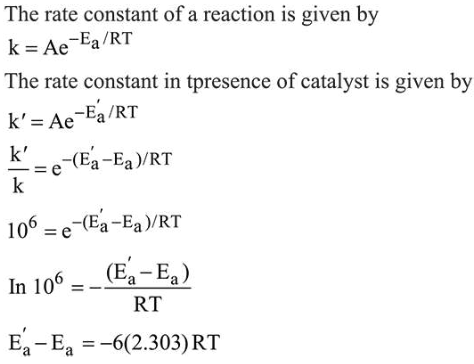# The rate of a certain biochemical reaction at physiological temperature (T)Question:

The rate of a certain biochemical reaction at physiological temperature $(T)$ occurs $10^{6}$ times faster with enzyme than without. The change in the activation energy upon adding enzyme is:

1. $-6(2.303) \mathrm{RT}$

2. $-6 R T$

3. $+6(2.303) \mathrm{RT}$

4. +6RT

Correct Option: 1

Solution: